### 4.12 Time Integration

This directory consists of the following primary files:

 TimeIntegrationScheme.h TimeIntegrationScheme.cpp TimeIntegrationGLM.h TimeIntegrationGLM.cpp *TimeIntegrationSchemes.h Specific GLM schemes, e.g. AB, RK, IMEX, etc. TimeIntegrationFIT.h TimeIntegrationFIT.cpp TimeIntegrationGEM.h TimeIntegrationGEM.cpp TimeIntegrationSDC.h TimeIntegrationSDC.cpp

The original time-stepping interface (found in the parent class TimeIntegrationScheme) where implemented by Vos et al.  and a detailed explanation of the mathematics and computer science concepts are provided there. After the original implementation, the time-stepping routines were refactored into a factory pattern (found in the child class TimeIntegrationGLM with specific GLM schemes covering both traditional and exponential schemes (compartmentalize in the *TimeIntegrationSchemes classes). Each GLM scheme sets up one or more (linear multistep methods) phases using the TimeIntegrationAlgorithmGLM class, which is where the GLM algorithm is performed using the helper class TimeIntegrationSolutionGLM.

More recently Extrapolation, Spectral-Deferred-Correction, and Fractional-in-time schemes have been added as sibbling classes to the GLM class (found in the child classes TimeIntegrationGEM, TimeIntegrationSDC, and TimeIntegrationFIT, respectively). The Extrapolation, Spectral-Deferred-Correction, and the Fractional-in-time schemes make use of their own separate algorithm.

#### 4.12.1 General Linear Methods

General linear methods (GLMs) can be considered as the generalization of a broad range of different numerical methods for ordinary differential equations. They were introduced by Butcher and they provide a unified formulation for traditional methods such as the Runge-Kutta methods and the linear multi-step methods such as Adams-Bashforth. From an implementation point of view, all these numerical methods can be abstracted similarly and allows a high level of generality which is the case in Nektar++. For background information about general linear methods, see .

##### Introduction

The standard initial value problem can written in the form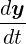= f(t,y), y(t0) = y0

where y is a vector containing the variable (or an array of array containing the variables).

In the formulation of general linear methods, it is more convenient to consider the ODE in autonomous form, i.e.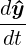= ^ f (^y ), ^ y (t0) = ^ y 0.

##### Formulation

Suppose the governing differential equation is given in autonomous form, the nth step of the GLM comprising

• r steps (as in a multi-step method)
• s stages (as in a Runge-Kutta method)

is formulated as:

 Y i = Δt∑ j=0s-1a ijFj + ∑ j=0r-1u ij^ y j[n-1], i = 0,1,…,s - 1 ^y i[n] = Δt∑ j=0s-1b ijFj + ∑ j=0r-1v ij^ y j[n-1], i = 0,1,…,r - 1

where Y i are referred to as the stage values and Fj as the stage derivatives. Both quantities are related by the differential equation:

 Fi = ^ f (Y i).

The matrices A = [aij], U = [uij], B = [bij], V = [vij] are characteristic of a specific method. Each scheme can then be uniquely defined by a partitioned (s + r) × (s + r) matrix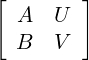##### Matrix notation

 ^y [n-1] =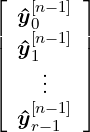, ^ y [n] =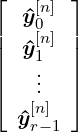, Y =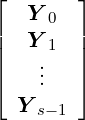, F =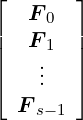the general linear method can be written more compactly in the following form: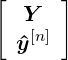=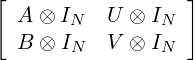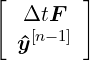where IN is the identity matrix of dimension N × N.

##### General Linear Methods in Nektar++

Although the GLM is essentially presented for ODE’s in its autonomous form, in Nektar++ it will be used to solve ODE’s formulated in non-autonomous form. Given the ODE,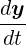= f(t,y), y(t0) = y0

a single step of GLM can then be evaluated in the following way:

• input

y[n-1], i.e. the r subvectors comprising yi[n-1] - t[n-1], i.e. the equivalent of y[n-1] for the time variable t.

• step 1: The stage values Y i, Ti and the stage derivatives Fi are calculated through the relations:
1. Y i = Δt∑ j=0s-1aijFj + ∑ j=0r-1uijyj[n-1], i = 0,1,…,s - 1
2. Ti  =  Δt∑ j=0s-1aij + ∑ j=0r-1uijtj[n-1], i = 0,1,…,s - 1
3. Fi  =  f(Ti,Y i), i = 0,1,…,s - 1
• step 2: The approximation at the new time level y[n] is calculated as a linear combination of the stage derivatives Fi and the input vector y[n-1]. In addition, the time vector t[n] is also updated

1. yi[n] = Δt∑ j=0s-1bijFj + ∑ j=0r-1vijyj[n-1], i = 0,1,…,r - 1
2. ti[n]  =  Δt∑ j=0s-1bij + ∑ j=0r-1vijtj[n-1], i = 0,1,…,r - 1
• output
1. y[n], i.e. the r subvectors comprising yi[n]. y0[n] corresponds to the actual approximation at the new time level.
2. t[n] where t0[n] is equal to the new time level t + Δt.

For a detailed description of the formulation and a deeper insight of the numerical method see .

##### Types of GLM time Integration Schemes

Nektar++ contains various classes and methods which implement the concept of GLMs. This toolbox is capable of numerically solving the generalised ODE using a broad range of different time-stepping methods. We distinguish the following types of general linear methods:

• Formally Explicit Methods: These types of methods are considered explicit from an ODE point of view. They are characterised by a lower triangular coefficient matrix A, ”i.e.” aij = 0 for j ≥ i. To avoid confusion, we make a further distinction:
• direct explicit method: Only forward operators are required.
• indirect explicit method: The inverse operator is required.
• Diagonally Implicit Methods: Compared to explicit methods, the coefficient matrix A has now non-zero entries on the diagonal. This means that each stage value depend on the stage derivative at the same stage, requiring an implicit step. However, the calculation of the different stage values is still uncoupled. Best known are the DIRK schemes.
• IMEX schemes: These schemes support the concept of being able to split right hand forcing term into an explicit and implicit component. This is useful in advection diffusion type problems where the advection is handled explicitly and the diffusion is handled implicit.
• Fully Implicit Methods: The coefficient matrix has a non-zero upper triangular part. The calculation of all stages values is fully coupled.
• Exponential Methods: A forward Euler method has been extended to the exponential setting. These types of methods are considered explicit from an ODE point of view. They are characterized by a coefficient matrix of exponential values for each variable. Two first order schemes have been implemented:
• Lawson-Euler explicit method: yn = φ0(z)N(yn-1,tn-1) + φ0(z)yn-1
• Nørsett-Euler explicit method: yn = φ1(z)N(yn-1,tn-1) + φ0(z)yn-1

where φ0(z) = ez for k = 0, φk(z) =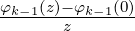for k ≥ 1.

While implemented, exponential schemes are not currently seeded.

The aim in Nektar++ is to fully support the first three types of GLMs. Fully implicit methods are currently not implemented.

#### 4.12.2 Extrapolation Integration Schemes

In addition to the GLMs Nektar++ also supports extrapolation methods:

• Extrapolation Methods: Extrapolation methods allow the construction of arbitrarily high-order time-integration methods based on a series for low-order approximations.

The Extrapolation methods follow the same paradigm as the GLMs, that is both have the same parent class and as such have the same interface.

For more details about Extrapolation methods, interested readers can refer to .

#### 4.12.3 Extrapolation Integration Schemes

In addition to the GLMs Nektar++ also supports extrapolation methods:

• Extrapolation Methods: Extrapolation methods allow the construction of arbitrarily high-order time-integration methods based on a series for low-order approximations.

The Extrapolation methods follow the same paradigm as the GLMs, that is both have the same parent class and as such have the same interface.

For more details about Extrapolation methods, interested readers can refer to .

#### 4.12.4 Spectral Deferred Correction Integration Schemes

In addition to the GLMs Nektar++ also supports spectral deferred correction methods:

• Spectral Deferred Correction Methods: Spectral Deferred Correction methods allow the construction of arbitrarily high-order time-integration methods based on a series for low-order approximations.

The Spectral Deferred Correction methods follow the same paradigm as the GLMs, that is both have the same parent class and as such have the same interface.

For more details about Spectral Deferred Correction methods, interested readers can refer to .

#### 4.12.5 Fractional-in-time Integration Schemes

In addition to the GLMs Nektar++ also supports fractional methods:

• Fractional-in-time Methods: A fast convolution algorithm for computing solutions to (Caputo) time-fractional differential equations. This is an explicit solver that expresses the solution as an integral over a Talbot curve, which is discretized with quadrature. First-order quadrature is currently implemented (Soon be expanded to forth order).

The Fractional methods follow the same paradigm as the GLMs, that is both have the same parent class and as such have the same interface.

#### 4.12.6 Usage

The goal of abstracting the concept of general linear methods and fractional methods is to provide users with a single interface for time-stepping, independent of the chosen method. The classes tree allows the user to numerically integrate ODE’s using high-order complex schemes, as if it was done using the Forward-Euler method. Switching between time-stepping schemes is as easy as changing a parameter in an input file.

In the already implemented solvers the time-integration schemes have been set up according to the nature of the equations. For example the incompressible Navier-Stokes equations solver allows the use of three different Implicit-Explicit time-schemes if solving the equations with a splitting-scheme. This is because this kind of scheme has an explicit and an implicit operator that combined solve the ODE’s system.

Once aware of the problem’s nature and implementation, the user can easily switch between some (depending on the problem) of the following time-integration schemes:

Note: in many cases the first order schemes reduce to either a forward or backward Euler scheme.

Nektar++ input file for your problem will require a string corresponding to the time-stepping method and the order, and optionally the variant and free parameters. In addition, parameters that define your integration in time, the time-step and number of steps or final time. For example:

1 <TIMEINTEGRATIONSCHEME>
2   <METHOD> IMEX </METHOD>
3   <VARIANT> DIRK </VARIANT>
4   <ORDER> 2 </ORDER>
5   <FREEPARAMETERS> 2 3 </FREEPARAMETERS>
6 </TIMEINTEGRATIONSCHEME>
7
8 <SOLVERINFO>
10   <I PROPERTY="Projection"            VALUE="DisContinuous"               />
14   <I PROPERTY="UpwindType"            VALUE="Upwind"                      />
15 </SOLVERINFO>
16
17 <PARAMETERS>
18   <P> TimeStep      = 0.0005              </P>
19   <P> NumSteps      = 200                 </P>
20   <P> wavefreq      = PI                  </P>
21   <P> epsilon       = 1.0                 </P>
22   <P> ax            = 2.0                 </P>
23   <P> ay            = 2.0                 </P>
24</PARAMETERS>

The old schema which specified the method, variant, order, and free parameters as a single string has been deprecated but remains backwards compatible.

#### 4.12.7 Implementation of a time-dependent problem

In order to implement a new solver which takes advantage of the time-integration class in Nektar++, two main ingredients are required:

• A main files in which the time-integration of you ODE’s system is initialized and performed.
• A class representing the spatial discretization of your problem, which reduces your system of PDE’s to a system of ODE’s.

Your pseudo-main file, where you actually loop over the time steps, will look like

1NekDouble timestep    = 0.1;
2NekDouble time        = 0.0;
3int NumSteps          = 1000;
4
5YourClass solver(Input);
6
7LibUtilities::TimeIntegrationScheme  TIME_SCHEME;
8LibUtilities::TimeIntegrationSchemeOperators ODE;
9
10ODE.DefineOdeRhs(&YourClass::YourExplicitOperatorFunction,solver);
11ODE.DefineProjection(&YourClass::YourProjectionFunction,solver);
12ODE.DefineImplicitSolve(&YourClass::YourImplicitOperatorFunction,solver);
13
14Array<OneD, LibUtilities::TimeIntegrationSchemeSharedPtr> IntScheme;
15
16LibUtilities::TimeIntegrationSolutionSharedPtr ode_solution;
17
18Array<OneD, Array<OneD, NekDouble> > U;
19
20TIME_SCHEME = LibUtilities::eForwardEuler;
21int numMultiSteps=1;
22IntScheme = Array<OneD,LibUtilities::TimeIntegrationSchemeSharedPtr>(numMultiSteps);
23LibUtilities::TimeIntegrationSchemeKey IntKey(TIME_SCHEME);
24IntScheme = LibUtilities::TimeIntegrationSchemeManager()[IntKey];
25ode_solution = IntScheme->InitializeScheme(timestep,U,time,ODE);
26
27for(int n = 0; n < NumSteps; ++n) {
28    U = IntScheme->TimeIntegrate(timestep,ode_solution,ODE);
29}

We can distinguish three different sections in the code above

##### Definitions
1NekDouble timestep    = 0.1;
2NekDouble time        = 0.0;
3int NumSteps          = 1000;
4
5YourClass equation(Input);
6
7LibUtilities::TimeIntegrationScheme  TIME_SCHEME;
8LibUtilities::TimeIntegrationSchemeOperators ODE;
9
10ODE.DefineOdeRhs(&YourClass::YourExplicitOperatorFunction,equation);
11ODE.DefineProjection(&YourClass::YourProjectionFunction, equation);
12ODE.DefineImplicitSolve(&YourClass::YourImplicitOperatorFunction, equation);

In this section you define the basic parameters (like time-step, initial time, etc.) and the time-integration objects. The operators are not all required, it depends on the nature of your problem and on the type of time integration schemes you want to use. In this case, the problem has been set up to work just with Forward-Euler, then for sure you will not need the implicit operator. An object named `equation` has been initialized, is an object of type `YourClass`, where your spatial discretization and the functions which actually represent your operators are implemented. An example of this class will be shown later in this page.

##### Initialisations
1Array<OneD,LibUtilities::TimeIntegrationSchemeSharedPtr> IntScheme;
2
3LibUtilities::TimeIntegrationSolutionSharedPtr ode_solution;
4
5Array<OneD, Array<OneD, NekDouble> > U;
6
7TIME_SCHEME = LibUtilities::eForwardEuler;
8int numMultiSteps=1;
9IntScheme = Array<OneD,LibUtilities::TimeIntegrationSchemeSharedPtr>(numMultiSteps);
10LibUtilities::TimeIntegrationSchemeKey IntKey(TIME_SCHEME);
11IntScheme = LibUtilities::TimeIntegrationSchemeManager()[IntKey];
12ode_solution = IntScheme->InitializeScheme(timestep,U,time,ODE);

The second part consists in the scheme initialization. In this example we set up just Forward-Euler, but we can set up more then one time-integration scheme and quickly switch between them from the input file. Forward-Euler does not require any other scheme for the start-up procedure. High order multi-step schemes may need lower-order schemes for the start up.

##### Integration
1for(int n = 0; n < NumSteps; ++n) {
2    U = IntScheme->TimeIntegrate(timestep,ode_solution,ODE);
3}

The last step is the typical time-loop, where you iterate in time to get your new solution at each time-level. The solution at time tn+1 is stored into vector `U` (you need to properly initialize this vector). `U` is an Array of Arrays, where the first dimension corresponds to the number of variables (eg. u,v,w) and the second dimension corresponds to the variables size (e.g. the number of modes or the number of physical points).

The variable `ODE` is an object which contains the methods. A class representing a PDE equation (or a system of equations) must have a series of functions representing the implicit/explicit part of the method, which represents the reduction of the PDE’s to a system of ODE’s. The spatial discretization and the definition of this method should be implemented in `YourClass`. `&YourClass::YourExplicitOperatorFunction` is a functor, i.e. a pointer to a function where the method is implemented. `equation` is a pointer to the object, i.e. the class, where the function/method is implemented. Here a pseudo-example of the .h file of your hypothetical class representing the set of equations. The implementation of the functions is meant to be in the related .cpp file.

1class YourCalss
2
3public:
4    YourClass(INPUT);
5
6    ~YourClass(void);
7
8    void YourExplicitOperatorFunction(
9        const Array<OneD, Array<OneD, NekDouble> > & inarray,
10              Array<OneD, Array<OneD, NekDouble> > & outarray,
11        const NekDouble time);
12
13    void YourProjectionFunction(
14        const Array<OneD, Array<OneD, NekDouble> > & inarray,
15              Array<OneD, Array<OneD, NekDouble> > & outarray, const
16              NekDouble time);
17
18    void YourImplicitOperatorFunction(
19        const Array<OneD, Array<OneD, NekDouble> > & inarray,
20              Array<OneD, Array<OneD, NekDouble> > & outarray, const
21              NekDouble time,
22        const NekDouble lambda);
23
24    void InternalMethod1(...);
25
26    NekDouble internalvariale1;
27
28protected:
29    ...
30
31private:
32    ...
33};

#### 4.12.8 Strongly imposed essential boundary conditions

Dirichlet boundary conditions can be strongly imposed by lifting the known Dirichlet solution. This is equivalent to decompose the approximate solution y into an known part, yD, which satisfies the Dirichlet boundary conditions, and an unknown part, yH, which is zero on the Dirichlet boundaries, i.e.

 y = yD + yH

In a Finite Element discretisation, this corresponds to splitting the solution vector of coefficients y into the known Dirichlet degrees of freedom yD and the unknown homogeneous degrees of freedom yH. Ordering the known coefficients first, this corresponds to:

 y =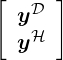The generalised formulation of the general linear method (i.e. the introduction of a left hand side operator) allows for an easier treatment of these types of boundary conditions. To better appreciate this, consider the equation for the stage values for an explicit general linear method where both the left and right hand side operator are linear operators, i.e. they can be represented by a matrix.

 MY i = Δt∑ j=0i-1a ijLY j + ∑ j=0r-1u ijyj[n-1], i = 0,1,…,s - 1

In case of a lifted known solution, this can be written as: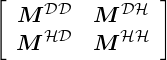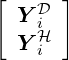= Δt∑ j=0i-1a ij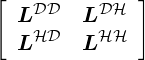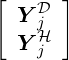+ ∑ j=0r-1u ij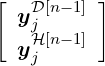, i = 0,1,…,s - 1

In order to calculate the stage values correctly, the explicit operator should now be implemented to do the following: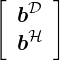=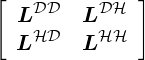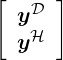Note that only the homogeneous part bH will be used to calculate the stage values. This means essentially that only the bottom part of the operation above, i.e. LHDyD + LHHyH is required. However, sometimes it might be more convenient to use/implement routines for the explicit operator that also calculate bD.

An implicit method should solve the system: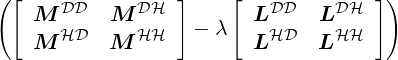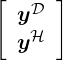=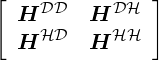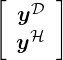=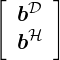for the unknown vector y. This can be done in three steps:

• Set the known solution yD
• Calculate the modified right hand side term bH-HHDyD
• Solve the system below for the unknown yH, i.e. HHHyH = bH-HHDyD

#### 4.12.9 How to add a new GLM time-stepping method

To add a new GLM time integration scheme, follow the steps below:

• Choose a name for the method, and create a header starting with the method name followed by `TimeIntegrationScheme.h`. Add the header file to the `SchemeInitializor.cpp` and register the method name with the factory, via REGISTER.
• Add the header file of the `CmakeLists.txt` in the parent directory.
• In the header file create a new class starting with the method name followed by `TimeIntegrationScheme`. The class must be able to handle any variants, orders, and free parameters. A simple example is in `EulerTimeIntegrationSchemes.h` which has two variants, Forward and Backward. In general the class must have the following methods:
• Constructor - creates the number of phases required and set the scheme data via a call to `SetupSchemeData`.
• `GetName` - returns the method name.
• `GetTimeStability` - returns time stability.
• `SetupSchemeData` - sets up the Butcher tableau for each phase of the sheme (TimeIntegrationAlgorithmGLM).
• Add documentation for the method (especially indicating what the auxiliary parameters of the input and output vectors of the multi-step method represent)

#### 4.12.10 Examples of already implemented time stepping schemes

What follows are some examples time-stepping schemes currently implemented in Nektar++, to give an idea of what is required to add one of them.

##### Forward Euler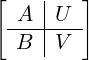=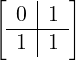, y[n] =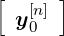=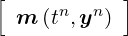##### Backward Euler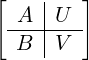=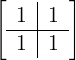, y[n] ==##### 2nd order Adams-Bashforth compact form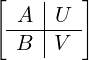=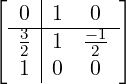, y[n] =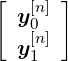=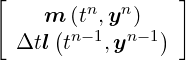##### 2nd order Adams-Bashforth classic form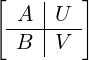=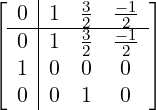, y[n] =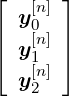=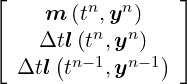Note: Adams-Bashforth and other explicit methods can be writen using a compact Bucther Tableau that reduces the number of steps. Throughout Nektar++ this compact form is utilized. This classic form is shown for educational purposes only.

##### 1st order IMEX Euler-Backward/ Euler-Forward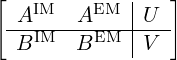=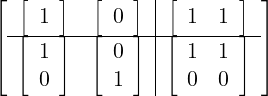with y[n] ==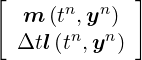##### 2nd order IMEX Backward Different Formula & Extrapolation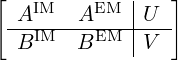=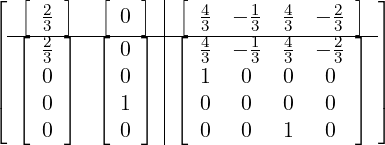with

 y[n] =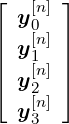=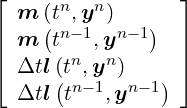Note: The first two rows are normalised so the coefficient on yn[n+1] is one. In the standard formulation it is 3∕2.

##### 3rdorder IMEX Backward Different Formula & Extrapolation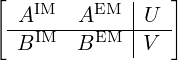=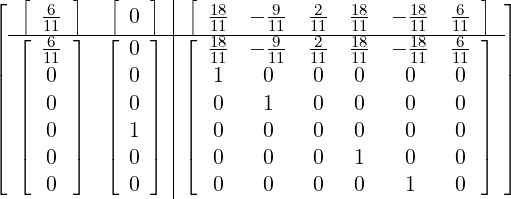with

 y[n] =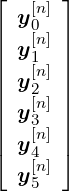=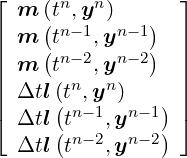Note: The first two rows are normalised so the coefficient on yn[n+1] is one. In the standard formulation it is 11∕6.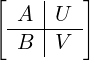=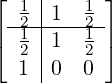, y[n] =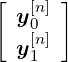=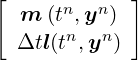##### Midpoint Method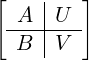=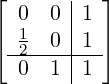, y[n] =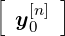=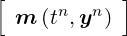##### RK4: the standard fourth-order Runge-Kutta scheme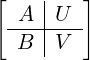=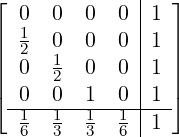, y[n] =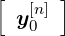=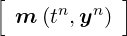##### 2nd order Diagonally Implicit Runge-Kutta (DIRK)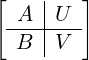=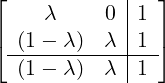with λ =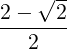, y[n] =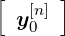=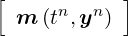##### 3rd order Diagonally Implicit Runge-Kutta (DIRK)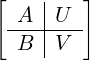=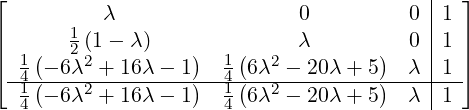with

 λ = 0.4358665215, y[n] =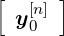=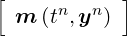##### 3rd order L-stable, three stage IMEX DIRK(3,4,3)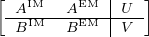=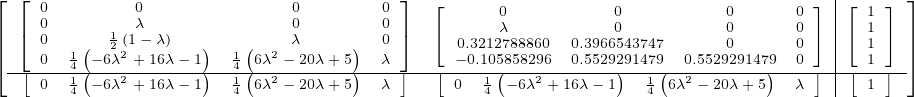with

 λ = 0.4358665215, y[n] ==##### 1st order Lawson Euler Exponential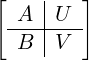=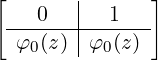, y[n] =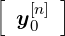=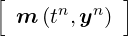##### 1st order Nørsett Euler Exponential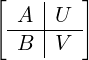=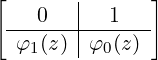, y[n] =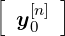=Note: The phi functions, φ0(z) and φ1(z) are defined as part of the discussion of GLM in Section 4.12.1.